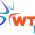Friday, 8 November 2013

Variable declaration in Razor in MVC

Variable declaration in Razor in MVC

As I discussed in my last article that Razor is the combination of HTML and C#, so we can use almost all programming feature in Razor.

In this article I am showing how we can work with variables.

In Razor we start programming code using @.

For Example:-

Create controller name Home and view Index

In this example I declared variable and calculate sum and print it.

Code of Index View

@{
Layout = null;
}

<!DOCTYPE html>

<html>
<meta name="viewport" content="width=device-width" />
<title>Index</title>
<body>

@{

int num1 = 10, num2 = 20;
int num3 = num1 + num2;

}

The sum of @num1 and @num2 is @num3

</body>
</html>

As you can see that I declared block using @ and inside this block I have declared variable and perform some calculation. After that closing the block I used these variables.

The output of this code as follows:-

Figure 1

Use of HTML code With C#

The best part of Razor is that we can put programming code with HTML in Razor.

For example:-

In the above example I put all variable result in <b> tag which is used to make text bold.

@{
Layout = null;
}

<!DOCTYPE html>

<html>
<meta name="viewport" content="width=device-width" />
<title>Index</title>
<body>

@{

int num1 = 10, num2 = 20;
int num3 = num1 + num2;

}

The sum of <b> @num1 </b> and <b>@num2</b> is <b>@num3</b>

</body>
</html>

Now see the output:-

Figure 2

For any query send your mail at info@techaltum.com

1.2.